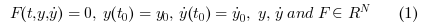Change language to:
English - Français - 日本語 - Português -

See the recommended documentation of this function

Справка Scilab >> Xcos > Solvers > IDA

# IDA

IDA (short for Implicit Differential Algebraic equations system solver) is a numerical solver providing an efficient and stable method to solve Differential Algebraic Equations system (DAEs) Initial Value Problems.

### Description

Called by xcos, IDA (short for Implicit Differential Algebraic equations system solver) is a numerical solver providing an efficient and stable method to solve Initial Value Problems of the form:Before solving the problem, IDA runs an internal routine to find consistent values for y0 and yPrime0 .

Starting then with those y0 and yPrime0 , IDA approximates yn+1 with the BDF formula:with, like in CVode, yn the approximation of y(tn) , hn = tn - tn-1 the step size, and the coefficients are fixed, uniquely determined by the method type, its order q ranging from 1 to 5 and the history of the step sizes.

Injecting this formula in (1) yields the system:To apply Newton iterations to it, we rewrite it into:with J an approximation of the Jacobian:α changes whenever the step size or the method order varies.

An implemented direct dense solver is used and we go on to the next step.

IDA uses the history array to control the local error yn(m) - yn(0) and recomputes hn if that error is not satisfying.

The function is called in between activations, because a discrete activation may change the system.

Following the criticality of the event (its effect on the continuous problem), we either relaunch the solver with different start and final times as if nothing happened, or, if the system has been modified, we need to "cold-restart" the problem by reinitializing it anew and relaunching the solver.

Averagely, IDA accepts tolerances up to 10-11. Beyond that, it returns a Too much accuracy requested error.

### Example

The 'Modelica Generic' block returns its continuous states, we can evaluate them with IDA by running the example: